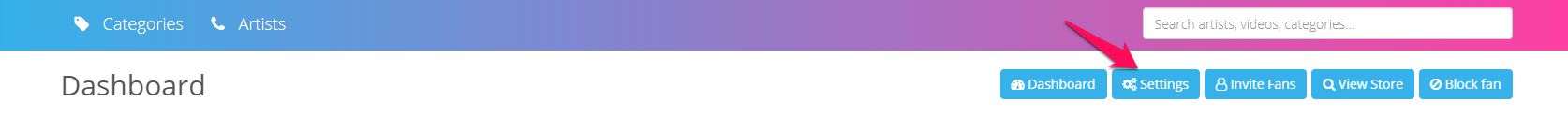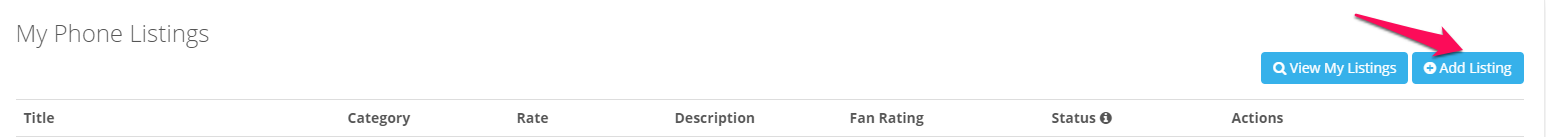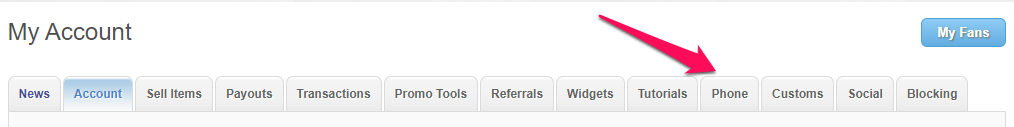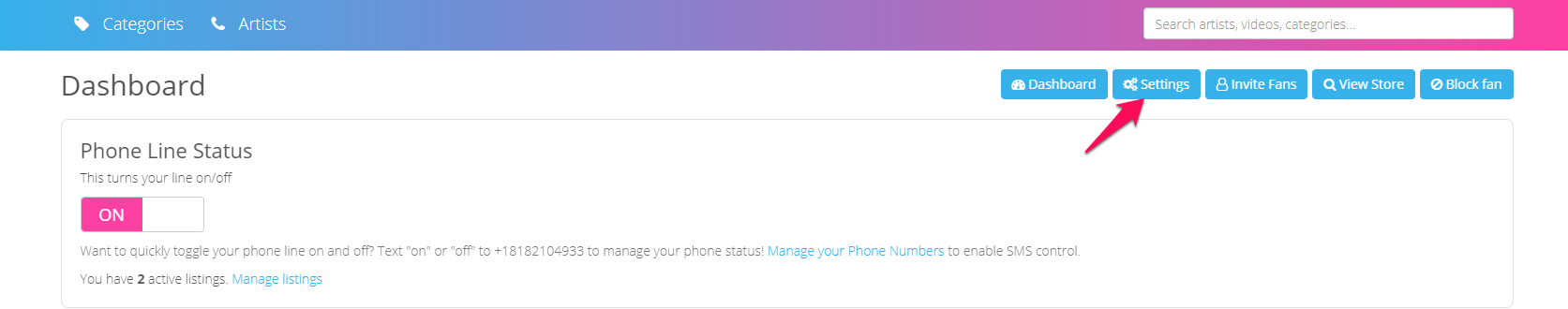To add a Phone Listing to iWantPhone:

1. Click on "Phone" within the iWantClips Artist Dashboard.2. Click on the "Settings" button in the upper, right-hand corner.3. Under "My Phone Listings", click on the "Add Listing" button in the upper, right-hand corner.This will take you to a page in which you can add your Listing Details, including:

• Rate Per Minute
* A Calculate Earnings button is next to this, so you can ensure you are receiving the per-minute rate of your choice.
• Type of Listing
• Listing Category
• Title
• Description
• Available Time Blocks
* 5, 10, 15, 20, 25, 30, 35, 40, 45, 50, 55, 60 minute blocks are available.

Verifying a Phone Number

To verify a phone number on iWantPhone:

To verify a phone number you must first be sure to add a phone listing to your store.

1. Click on "Phone" within the iWantClips Artist Dashboard.2. Click on the "Settings" button in the upper, right-hand corner.3. Scroll down to "My Phone Numbers" and click the "Add a Phone Number" button.Once you've added your phone number, you will be prompted to verify it, using a 4 digit pin.

Click the blue "Call To Verify" button to continue verifying. You will be called and then prompted to enter your pin. After doing so, you will see your number go from a yellow "Unverified" badge, to no badge indicator.

Country Codes for Adding a phone number:

• AC= Ascension Island (UK)
• AE= United Arab Emirates
• AF= Afghanistan
• AG= Antigua and Barbuda
• AI= Anguilla
• AL= Albania
• AM= Armenia
• AO= Angola
• AR= Argentina
• AS= American Samoa
• AT= Austria
• AU= Australia
• AW= Aruba
• AX= Aland Islands
• AZ= Azerbaijan
• BA= Bosnia and Herzegovina
• BE= Belgium
• BF= Burkina Faso
• BG= Bulgaria
• BH= Bahrain
• BI= Burundi
• BJ= Benin
• BL= Saint Barthelemy
• BM= Bermuda
• BN= Brunei Darussalam
• BO= Bolivia
• BQ= Bonaire
• BR= Brazil
• BS= Bahamas
• BT= Bhutan
• BW= Botswana
• BY= Belarus
• BZ= Belize
• CC= Cocos Islands
• CD= Congo
• CF= Central African Republic
• CG= Congo
• CH= Switzerland
• CI= Cote d’lvoire
• CK= Cook Islands
• CL= Chile
• CM= Cameroon
• CN= China
• CO= Colombia
• CR= Costa Rica
• CU= Cuba
• CV= Cabo Verde
• CW= Curacao
• CX= Christmas Island
• CY= Cyprus
• CZ= Czechia
• DE= Germany
• DJ= Djibouti
• DK= Denmark
• DM=Dominica
• DO= Dominican Republic
• DZ= Algeria
• EE= Estonia
• EG= Egypt
• EH= Western Sahara
• ER= Eritrea
• ES= Spain
• ET= Ethiopia
• FI= Finland
• FJ= Fiji
• FK= Falkland Islands
• FM= Micronesia
• FO= Faroe Islands
• FR= France
• GA= Gabon
• GB= Great Britain
• GE= Georgia
• GF= French Guiana
• GG= Guernsey
• GH= Ghana
• GI= Gibraltar
• GL= Greenland
• GM= Gambia
• GN= Guinea
• GQ= Equatorial Guinea
• GR= Greece
• GT= Guatemala
• GU= Guam
• GW= Guinea-Bissau
• GY= Guyana
• HK= Hong Kong
• HN= Honduras
• HR= Croatia
• HT= Haiti
• HU= Hungary
• ID= Indonesia
• IE= Ireland
• IL= Israel
• IM= Isle of Man
• IN= India
• IO= British Indian Ocean Territory
• IQ= Iraq
• IR= Iran
• IS= Iceland
• IT= Italy
• JE= Jersey
• JM= Jamaica
• JO= Jordan
• JP= Japan
• KE= Kenya
• KG= Kyrgyzstan
• KH= Cambodia
• KI= Kiribati
• KM= Comoros
• KN= Saint Kitts and Nevis
• KP= Democratic People’s Republic of Korea
• KR= Republic of Korea
• KW= Kuwait
• KY= Cayman Islands
• KZ= Kazakhstan
• LA= Lao
• LB= Lebanon
• LC= Saint Lucia
• LI= Liechtenstein
• LK= Sri Lanka
• LR= Liberia
• LS= Lesotho
• LT= Lithuania
• LU= Luxembourg
• LV= Latvia
• LY= Libya
• MA= Morocco
• MC= Monaco
• MD= Moldova
• ME= Montenegro
• MF= Saint Martin
• MH= Marshall Islands
• MK= Macedonia
• ML= Mali
• MM= Myanmar
• MN= Mongolia
• MO= Macau
• MP= Northern Mariana Islands
• MQ= Martinique
• MR= Mauritania
• MS= Montserrat
• MT= Malta
• MU= Mauritius
• MV= Maldives
• MW= Malawi
• MX= Mexico
• MY= Malaysia
• MZ= Mozambique
• NA= Namibia
• NC= New Caledonia
• NE= Niger
• NF= Norfolk Island
• NG= Nigeria
• NI= Nicaragua
• NL= Netherlands
• NO= Norway
• NP= Nepal
• NR= Nauru
• NU= Niue
• NZ= New Zealand
• OM= Oman
• PA= Panama
• PE= Peru
• PF= French Polynesia
• PG= Papua New Guinea
• PH= Philippines
• PK= Pakistan
• PL= Poland
• PM= Saint Pierre and Miquelon
• PR= Puerto Rico
• PS= Palestine
• PT= Portugal
• PW= Palau
• PY= Paraguay
• QA= Qatar
• RE= Reunion
• RO= Romania
• RS= Serbia
• RU= Russia
• RW= Rwanda
• SA= Saudi Arabia
• SB= Solomon Islands
• SC= Seychelles
• SD= Sudan
• SE= Sweden
• SG= Singapore
• SH= Saint Helena
• SI= Slovenia
• SJ= Svalbard and Jan Mayen
• SK= Slovakia
• SL= Sierra Leone
• SM= San Marino
• SN= Senegal
• SO= Somalia
• SR= Suriname
• SS= South Sudan
• ST= Sao Tome and Principe
• SX= Sint Maarten
• SY= Syrian Arab Republic
• SZ= Swaziland
• TA= Tristan da Cunha
• TC= Turks and Caicos Islands
• TG= Togo
• TH= Thailand
• TJ= Tajikistan
• TK= Tokelau
• TL= Timor-Leste
• TM= Turkmenistan
• TN= Tunisia
• TO= Tonga
• TR= Turkey
• TV= Tuvalu
• TW= Taiwan
• TZ= Tanzania
• UA= Ukraine
• UG= Uganda
• US= United States of America
• UY= Uruguay
• UZ= Uzbekistan
• VA= Holy See
• VC= Saint Vincent and the Grenadines
• VE= Venezuela
• VG= British Virgin Islands
• VI= US Virgin Islands
• VN= Vietnam
• VU= Vanuatu
• WF= Wallis and Futuna
• WS= Samoa
• YE= Yemen
• YT= Mayotte
• ZA= South Africa
• ZM= Zambia
• ZW= Zimbabwe

If you ever have questions about iWantPhone, we are here to help! Please reach out to us anytime at Support@iWantClips.com

Thank you so much!